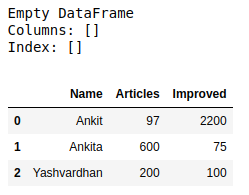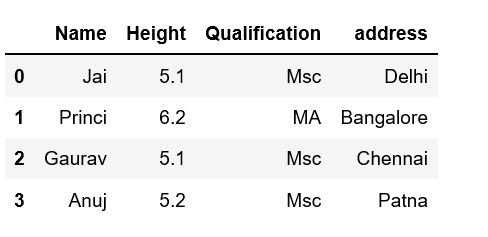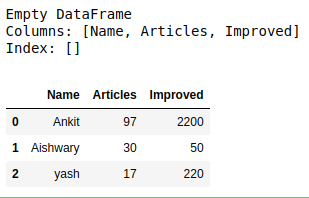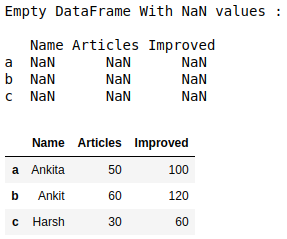# How to create an empty DataFrame and append rows & columns to it in Pandas?

• Last Updated : 14 Jul, 2022

Let’s discuss how to create an empty DataFrame and append rows & columns to it in Pandas n Python. There are multiple ways in which we can do this task. Here we will cover the following section:

• Creating an empty Dataframe in Pandas
• Append row to Dataframe in Pandas
• Append row to Dataframe in Pandas

## Python3`# import pandas library as pd``import` `pandas as pd` `# create an Empty DataFrame object``df ``=` `pd.DataFrame()` `print``(df)`

Output:

```Empty DataFrame
Columns: []
Index: []```

### Append Column to Dataframe

Example 1:

Create a complete empty DataFrame without any column name or indices and then append columns in Pandas one by one to it.

## Python3

 `# import pandas library as pd``import` `pandas as pd` `# create an Empty DataFrame object``df ``=` `pd.DataFrame()` `print``(df)` `# append columns to an empty DataFrame``df[``'Name'``] ``=` `[``'Ankit'``, ``'Ankita'``, ``'Yashvardhan'``]` `df[``'Articles'``] ``=` `[``97``, ``600``, ``200``]` `df[``'Improved'``] ``=` `[``2200``, ``75``, ``100``]` `df`

Output:Example 2:

This method will create a new Dataframe with a new column added to the old Dataframe using assign in Pandas.

## Python3

 `# Import pandas package``import` `pandas as pd` `# Define a dictionary containing Students data``data ``=` `{``'Name'``: [``'Jai'``, ``'Princi'``, ``'Gaurav'``, ``'Anuj'``],``    ``'Height'``: [``5.1``, ``6.2``, ``5.1``, ``5.2``],``    ``'Qualification'``: [``'Msc'``, ``'MA'``, ``'Msc'``, ``'Msc'``]}`  `# Convert the dictionary into DataFrame``df ``=` `pd.DataFrame(data)` `# Using 'Address' as the column name and equating it to the list``df2 ``=` `df.assign(address``=``[``'Delhi'``, ``'Bangalore'``, ``'Chennai'``, ``'Patna'``])` `# Observe the result``print``(df2)`

Output:### Append row to Dataframe

Example 1:

Create an empty DataFrame with columns name only then append rows one by one to it using append() method

## Python3

 `# import pandas library as pd``import` `pandas as pd` `# create an Empty DataFrame``# object With column names only``df ``=` `pd.DataFrame(columns ``=` `[``'Name'``, ``'Articles'``, ``'Improved'``])``print``(df)` `# append rows to an empty DataFrame``df ``=` `df.append({``'Name'` `: ``'Ankit'``, ``'Articles'` `: ``97``, ``'Improved'` `: ``2200``},``        ``ignore_index ``=` `True``)` `df ``=` `df.append({``'Name'` `: ``'Aishwary'``, ``'Articles'` `: ``30``, ``'Improved'` `: ``50``},``        ``ignore_index ``=` `True``)` `df ``=` `df.append({``'Name'` `: ``'yash'``, ``'Articles'` `: ``17``, ``'Improved'` `: ``220``},``      ``ignore_index ``=` `True``)` `df`

Output:Example 2:

Create an empty DataFrame with a column name and indices and then append rows one by one to it using the loc[] method.

## Python3

 `# import pandas library as pd``import` `pandas as pd` `# create an Empty DataFrame object With``# column names and indices``df ``=` `pd.DataFrame(columns ``=` `[``'Name'``, ``'Articles'``, ``'Improved'``],``        ``index ``=` `[``'a'``, ``'b'``, ``'c'``])` `print``(``"Empty DataFrame With NaN values : \n\n"``, df)` `# adding rows to an empty``# dataframe at existing index``df.loc[``'a'``] ``=` `[``'Ankita'``, ``50``, ``100``]``df.loc[``'b'``] ``=` `[``'Ankit'``, ``60``, ``120``]``df.loc[``'c'``] ``=` `[``'Harsh'``, ``30``, ``60``]` `df`

Output:My Personal Notes arrow_drop_up